# How To Get Absolute Value in Google Sheets

Absolute value is the distance between a number and zero. Since distance cannot be negative, an absolute value is always a positive number, so for example, the absolute value of 5 is 5 and the absolute value of -5 is also 5.

Finding absolute values ​​in Google Sheets can be useful for a variety of applications, but how do you do it without having to do it manually?

Fortunately, there are three easy ways to accomplish this task. In this article, I’ll walk you through three methods you can use to get absolute value in Google Sheets.

## Finding Absolute Value in Google Sheets

Finding absolute values ​​in Spreadsheets is as easy as using one of three methods: the ABS function, the SUMPRODUCT function, or converting negative numbers to positives.

Check out how to use these three methods below.

### Using the ABS function in Google Sheets

ABS is a function in Google Sheets that returns the absolute value of a number.

You can always convert negative numbers to positives manually, and that would work just fine if you’re just trying to get an absolute value for one or two cells. However, imagine you have a large spreadsheet with a table column containing 350 negative numbers.

Fortunately, Google Sheets includes an ABS feature so you can quickly get absolute values ​​for negative numbers without editing their cells. It’s a basic function that you can enter using this syntax: `=ABS(value)`. The ABS value can be a cell reference or a number. For example, follow these steps:

1. Open a blank spreadsheet in Google Sheets.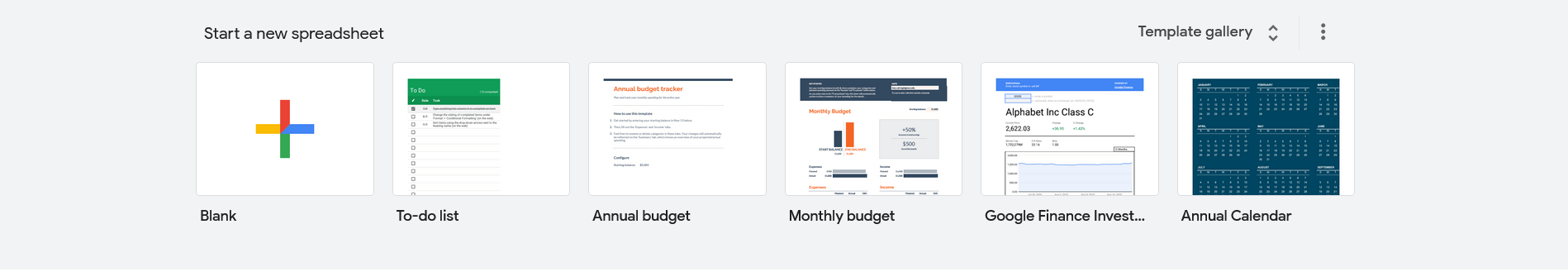2. Then enter the values ​​’-454′, ‘-250’ and -‘350’ in cells A2:A4 as shown in the snapshot directly below. Now you can convert that dummy data to absolute values.3. Select cell B2 and enter the function `=ABS(A2)` in the fx bar, and B2 returns the absolute value 454 when you press Enter.4. Copy the function to the other cells with the fill handle. Select B2, left click in the lower right corner of the cell and drag the cursor over B3 and B4. Then release the left mouse button to copy the ABS function to those cells as shown in the screenshot below.

ABS also calculates absolute values ​​for the results of calculations. For example, select B5, enter `=ABS(A2+A4)` in the function bar and press Return. B5 returns the absolute value of 804. The SUM function returns -804, but as an absolute value it returns 804.

### Using the SUMPRODUCT function in Google Sheets

ABS does not append a string of numbers within a single cell reference. In addition, a range of cells can contain a combination of positive and negative numbers. As such, a SUMPRODUCT combined with the ABS formula is perhaps the best way to add a series of numbers together to get an absolute value.

Before adding the SUMPRODUCT formula to your spreadsheet, type “200” in cell A5 and “300” in A6. Then enter the formula `=SUMPRODUCT(ABS(A2:A6))` in cell B6 and press Return. B6 now adds the cell range A2:A6 and returns an absolute value of 1,554.

You can also expand the formula to add two or more ranges of cells. Select cell B7 in your Sheets spreadsheet and enter the function `=SUMPRODUCT(ABS(A2:A6))+SUMPRODUCT(ABS(B2:B4))` in the function bar. The formula adds the numbers in the ranges A2:A6 and B2:B4 and then returns an absolute value total, which in this case is 2,608.

### Convert negative numbers to positive numbers

Power Tools is an add-on for Spreadsheets with tons of tools, including an option that converts license plates. To use this add-on, add Power Tools to Google Sheets and follow these instructions to convert negative numbers to positive numbers:3. Select Electrical tools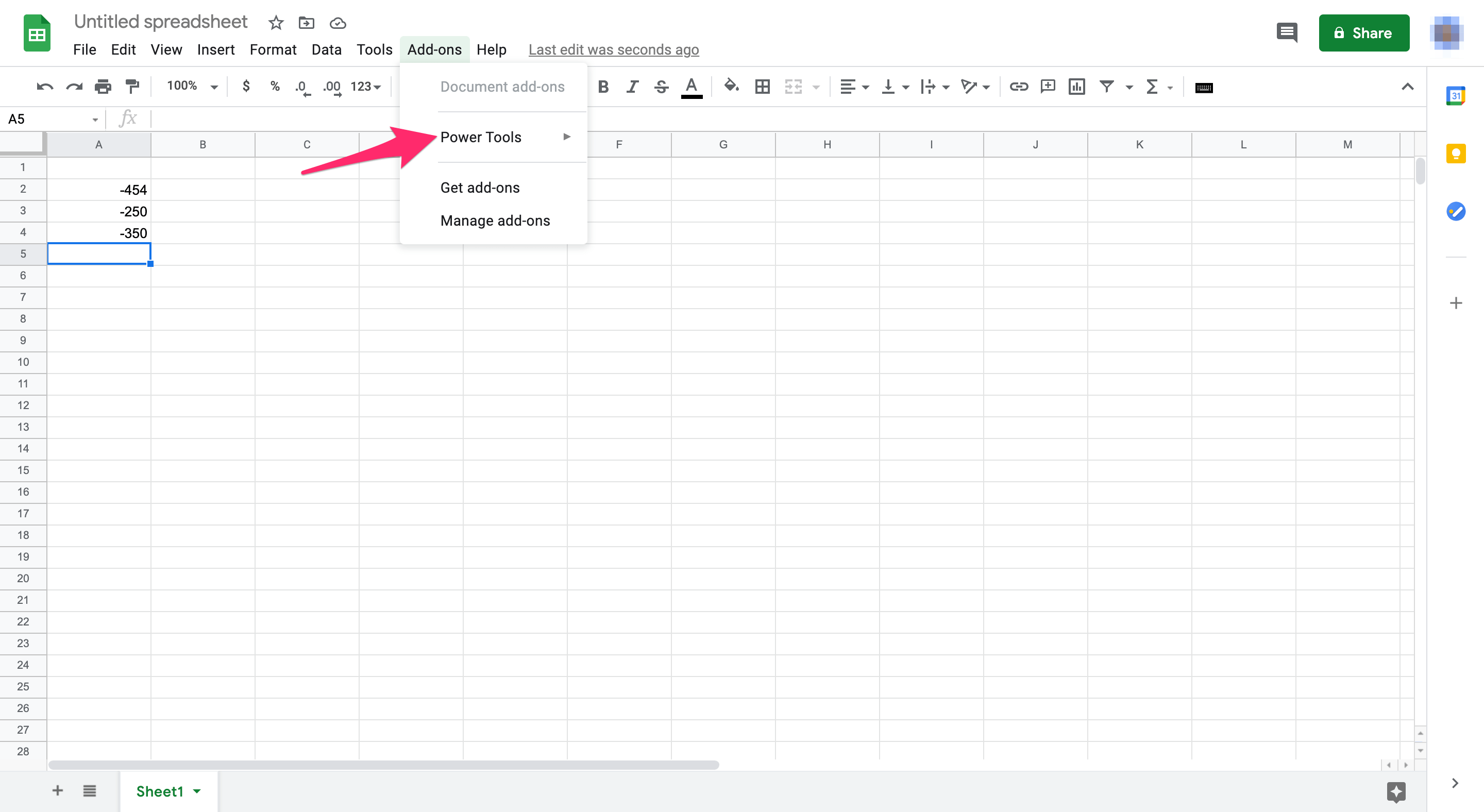4. Select Get started from the drop-down menu to open Power Tools as in the screenshot below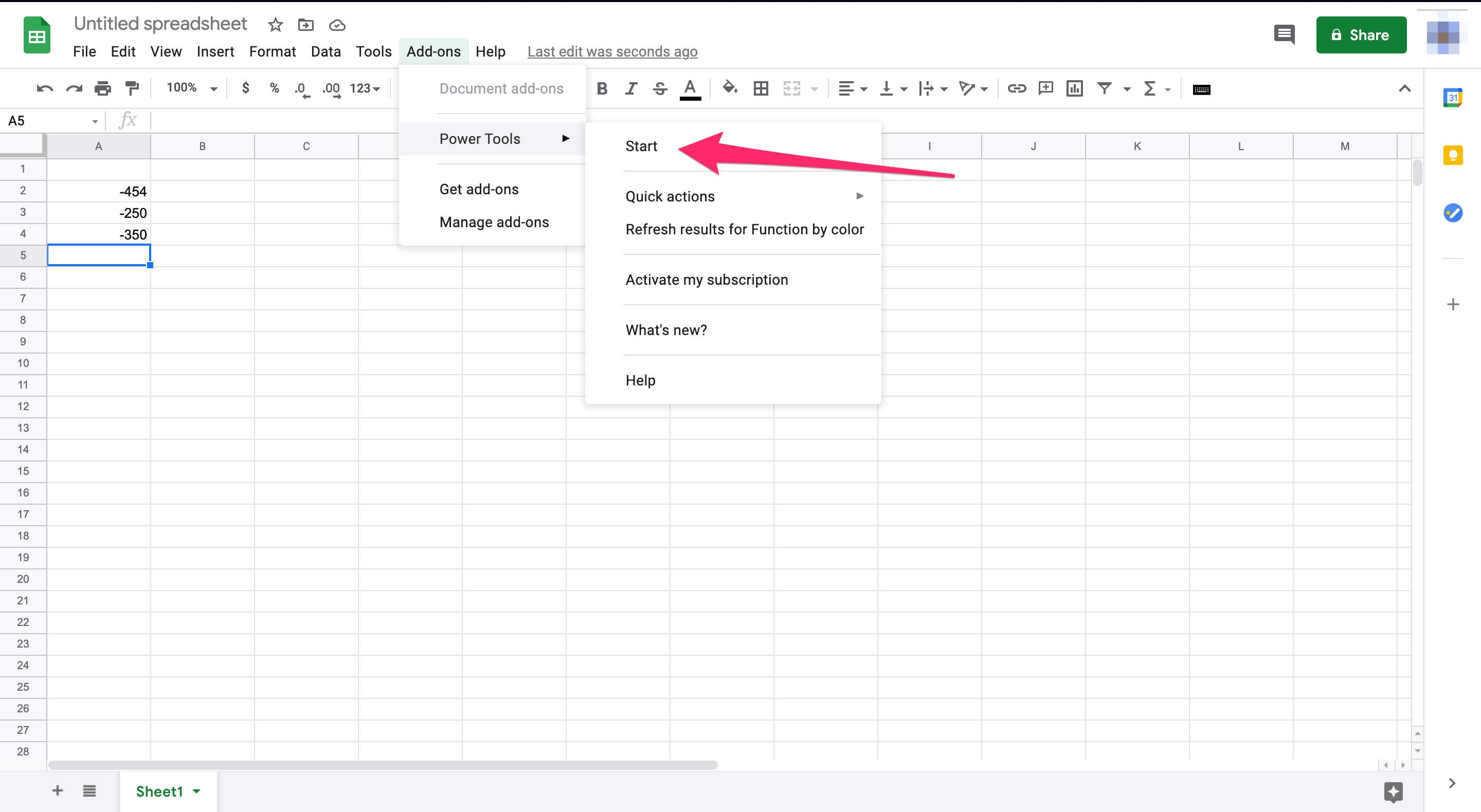5. Click transfer from the menu opened on the right6. Click on the Convert Number Sign checkbox.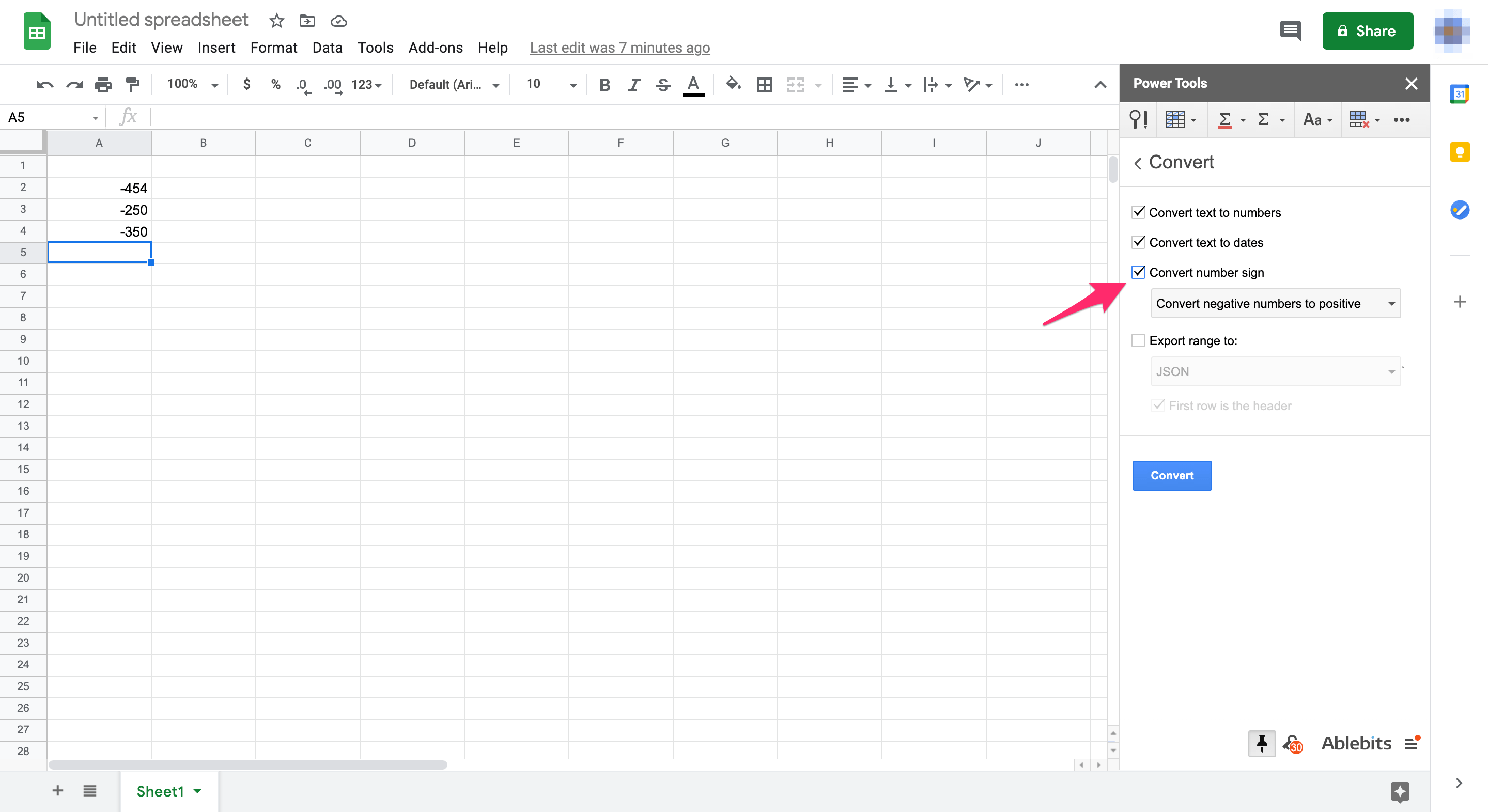7. Select Convert negative numbers to positive from the drop-down menu.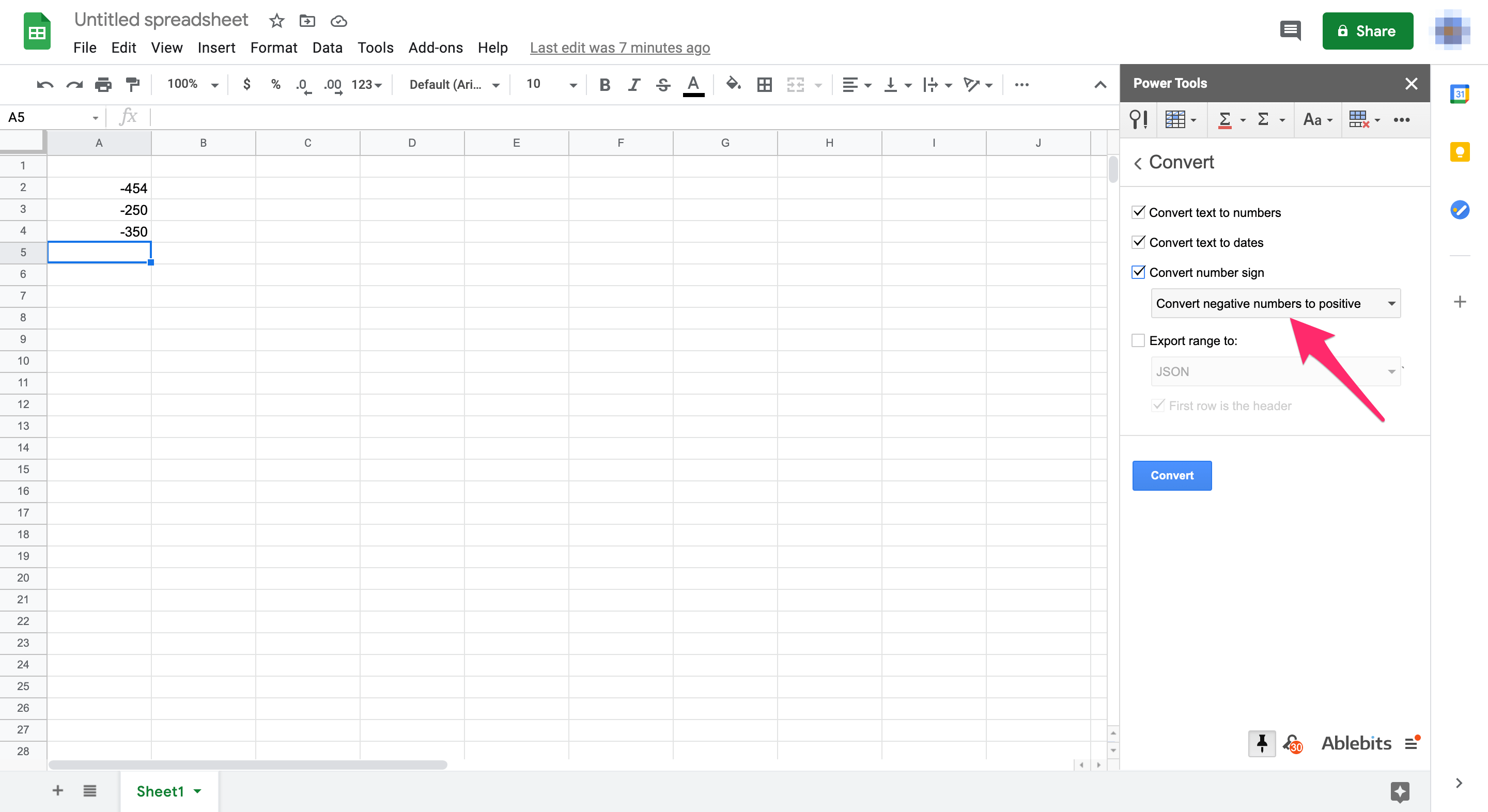8. Select the cell range A2:A4 on your Sheets spreadsheet with the cursor.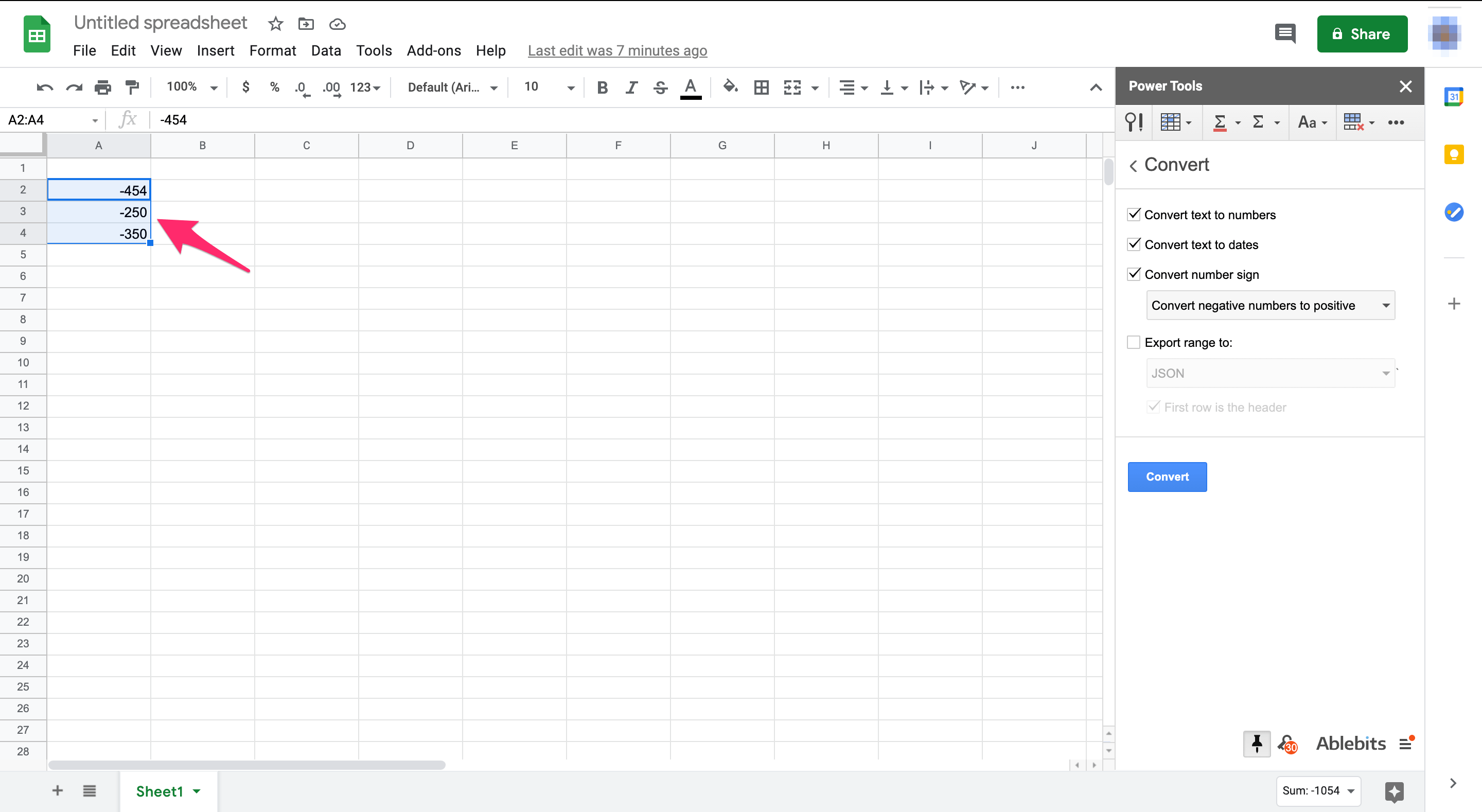9. Click on the transfer button in the add-ons sidebarThis process removes the negative characters from cells A2:A4 as shown in the screenshot below. Those cells now contain absolute values ​​instead of negative values. With this conversion option, you can quickly get the absolute values ​​for a large number of cells without entering an ABS function in an adjacent column. The Power Tools Add-on has become an essential tool for experienced Google Sheets users.

## Final Thoughts

By following any of the above methods, you can get absolute values ​​in Sheets without manually editing cells. If you are using Excel, it might be a useful tutorial to get Absolute value in Excel.

Do you have any Google Sheets tips and tricks that you find helpful? Comment below.